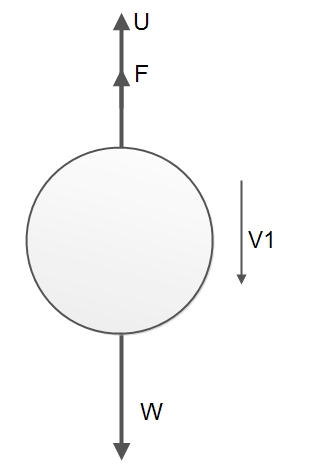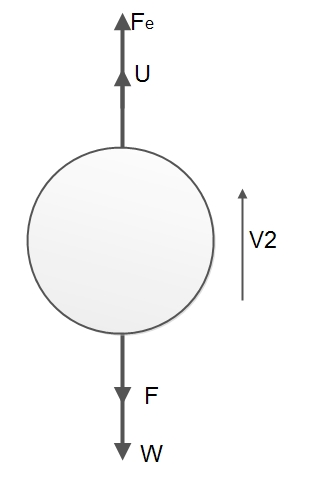Milikan's Oildrop Experiment1. It is based on Stoke's Law, i.e

"The force of viscosity on a small sphere moving through a viscous fluid is given by:where:

• Fd is the frictional force – known as Stokes' drag – acting on the interface between the fluid and the particle
• μ is the viscosity (sometimes "ita" η)
• R is the radius of the spherical object
• v is the flow velocity relative to the object."

In SI units, Fd is given in Newtons (= kg m s−2)
μ in Pa·s (= kg m−1 s−1)
R in meters
and v in m/s.

2. The apparatus consists of the doubled walled hollow apparatus where cold water is kept to maintain the constant temperature. The constant temperature must be maintained to maintain the constant coefficient of viscosity since the coefficient of viscosity is dependent on temperature.

3. The atomizer is kept to convert the large oil drop into tiny droplets. The large drops must be converted into tiny droplets because Stoke's law is not valid for the large drops. Simultaneously, a high electric field is required to maintain a large drop which is difficult to maintain.

4. Clock oil is used instead of other fluids to carry out the experiment. Because clock oil is the low-vapor pressure oil and reduces the problem of evaporation during the experiment. Moreover, clock oil gives quite small droplets which are required to attain terminal velocity soon after it is sprayed. If the water is used, small drops can't be obtained. As a result, Stoke's law can't be applied.

5. The apparatus consists of 3 windows. From the first window, visible rays are allowed to pass so that the tiny droplets can be seen. From the second window, X-rays are allowed to pass to ionize the medium so that the oil droplets get charged. And from the 3rd window, the microscope is inserted consisting of a calibrated lens to measure the distance traveled by the droplet.

6. The electric field must be varied constantly in the apparatus so that the oil droplet do not stick to the opposite plate. The electric field is varied by changing the voltage constantly with the help of rheostat. So, during this, the oil drop experiences the motion,
a. In the presence of gravity only.
b. In the presence of gravity and electric field both.

A. In the presence of gravity only:When oil drop moves between the plates it gets charged, let oil drop moves under gravity having free fall then it remains under the action of two different forces; force due to gravity (which acts vertically downward) and upthrust provided by air particle (which acts in the upward direction)
If the oil drop moves with constant velocity (Vg) also called terminal velocity then the net downward force is,Now, according to Stoke’s law, as the oil drop moves downward, viscous force (backward direction) is developed which is given byThen, in equilibrium,This gives the radius of oil drop.

B. In the presence of gravity and electric field both,if the electric field is applied, usually the negatively charged oil drop moves towards the positive plate. If the oil drop moves with a constant velocity ve. Then the force due to the electric field is equal to the net downward force.
Mathematically, it can be expressed as:At equilibrium condition equation 1 and 2 are equalNow putting the value of r in equation (iv) we getThis expression gives the charge of an oil drop. In Millikan's oil drop experiment, the value of q can be determined if the values of symbols are known.

ESTIMATION OF THE MASS OF AN ELECTRON:
If we assume that the electron which are the constituents of the cathode rays carry the fundamental charge as calculated by the milkman's oil-drop experiment. Then from Thomson's measured value of e/m, we can estimate the mass of electron.